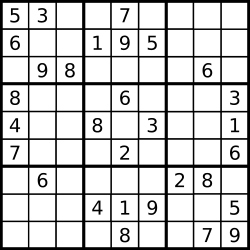# 37 Sudoku Solver

### Problem

Write a program to solve a Sudoku puzzle by filling the empty cells.

Empty cells are indicated by the character '.'.

You may assume that there will be only one unique solution.A sudoku puzzle......and its solution numbers marked in red.

### Solutions

``````public class Solution {
public void solveSudoku(char[][] board) {
solve(board, 0, 0);
}
private boolean solve(char[][] board, int i, int j){
if (i == board.length - 1 && j == board.length){
return true;
}
if (j == board.length){
i = i + 1;
j = 0;
}
if (board[i][j] == '.'){
for (int k = 1; k <= 9; k ++ ){
board[i][j] = (char)((int)'0' + k);
if (valid(board, i, j) == true){
boolean tmp = solve(board, i, j+1);
if (tmp == true){
return true;
}
}
}//for k
board[i][j] = '.';
}// i j
else{
return solve(board, i, j+1);
}

return false;
}
private boolean valid(char[][] board, int x, int y){
int check = 0;
for (int j = 0; j < board.length; j ++){
int val = board[x][j] - '0';
if (board[x][j] == '.'){
continue;
}
if ((check & (1<<(val-1))) == 1<<(val-1)){
return false;
}
else{
check = check | 1<<(val-1);
}
}
check = 0;
for (int i = 0; i < board.length; i ++){
int val = board[i][y] - '0';
if (board[i][y] == '.'){
continue;
}
if ((check & (1<<(val-1))) == 1<<(val-1)){
return false;
}
else{
check = check | 1<<(val-1);
}
}
int ib = x/3;
int jb = y/3;
check = 0;
for (int i = ib*3; i < (ib+1)*3; i ++){
for (int j = jb*3; j < (jb+1)*3; j++){
int val = board[i][j] - '0';
if (board[i][j] == '.'){
continue;
}
if ((check & (1<<(val-1))) == 1<<(val-1)){
return false;
}
else{
check = check | 1<<(val-1);
}
}
}

return true;
}
}
``````